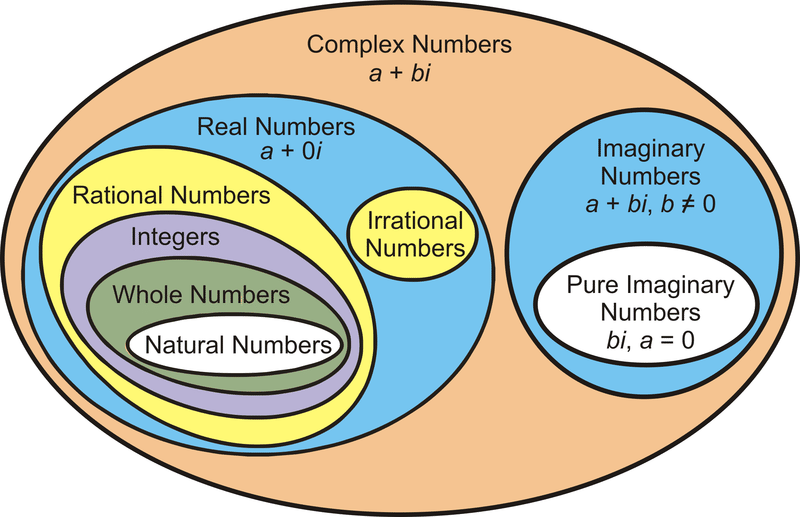2.3k views
What is the correct translation of the following statement into mathematical logic?

“Some real numbers are rational”

1. $\exists x (real(x) \lor rational(x))$
2. $\forall x (real(x) \to rational(x))$
3. $\exists x (real(x) \wedge rational(x))$
4. $\exists x (rational(x) \to real(x))$

edited | 2.3k views

Meaning of each choices:

1. There exists a number which is either real or rational
2. If a number is real it is rational
3. There exists a number which is real and rational
4. There exists a number such that if it is rational, it is real

by
edited by
+1
@Arjun Sir: Can we write the statement as follows?
$\exists x( Real(x) \implies Rational(x) )$

In English: There exists an x such that, if x is real then it is rational.
+6
@Pratyush, No that is incorrect because implication is also true when the antecedent is false.

Some numbers might not be real, and since here no domain is specified we consider domain of all numbers be it an integer or real etc.

So, when some number is not real, say it is integer, your first part of implication becomes false and hence the whole implication becomes true which should not happen.
0
what is the problem in option d,

it also conveys the same meaning i guess;

there exists a number such that if it is rational then real.

and question also says that some real numbers are rational numbers.

where am i wrong??
0

I get confused when to translate a statement as AND (^) and when to use implication (-->) .

eg. None of my friends are  perfect... here should i consider Friends ^ Perfect  or Friends-->Perfect

For statement : Good mobile phones are not cheap.  (from gate 2014) i used implication and that leads to the right answer.

Similarly Not all that glitters is gold(2014) also implication gives the correct result

Any tips or any source to refer pls

Translation of (C):" There exists a number which is real and rational " and this is eqt to “Some real numbers are rational”.

“ There exists a number which is real and rational” and this is equal to “Some real number are rational number.”edited
+1Rational numbers are always real